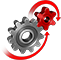# WolframSystemModeler

## An Influenza Epidemic

Using the System Dynamics library in Wolfram System Modeler, it is possible to model all kinds of systems of mass and information flows. In this example, we use the library to model the spread of an influenza virus in a population.

### The Influenza Model

The influenza model is mainly based on flows and rates, such as the rate of infection or the rate by which people recover from the flu. It does not presume any preventive actions, such as vaccination, so the model can be seen as the scenario when there is no intervention and the outbreak is left to run its course.The image shows the model as seen in the diagram view in Wolfram System Modeler. The model is almost solely composed of levels, rates, and simple math functions such as addition and division, but still manages to explain and simplify the complex system that an influenza outbreak is.

### Simulation ResultsThe initial non-infected population drastically drops as the influenza infects more and more people. How steep the drop is and how many people are infected depend, among other factors, on the contraction constant in the model. As can be seen, more than 90% of the population become infected, and so we are dealing with a highly contagious influenza brand. As the outbreak runs its natural course, the level of immunity increases, and within approximately 30 weeks, the epidemic is over.

Explore the System Dynamics library

Use the System Dynamics library in System Modeler to model an outbreak of an infectious disease.

## Wolfram System Modeler

Questions? Comments? Contact a Wolfram expert »